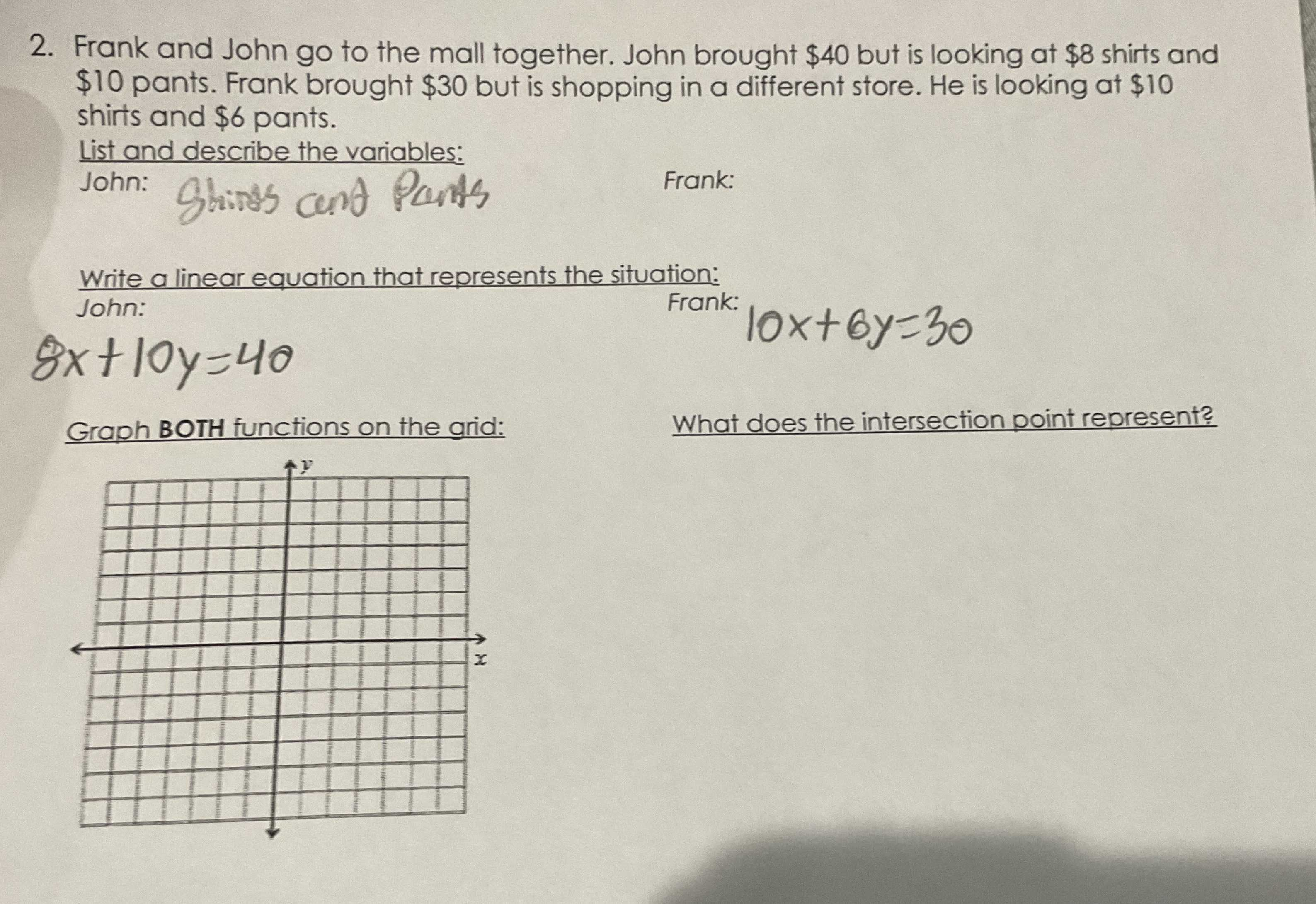### Still have math questions?

Algebra
Question2. Frank and John go to the mall together. John brought $$\ 40$$ but is looking at $$\ 8$$ shirts and

$$\ 10$$ pants. Frank brought $$\ 30$$ but is shopping in a different store. He is looking at $$\ 10$$ shirts and $$\ 6$$ pants.

List and describe the variables:

John:                   Frank:

Write a linear equation that represents the situation:

John:                   Frank:

Graph BOTH functions on the grid:              What does the intersection point represent?

$$8s+ 10p= 40\\10s+ 6p= 30$$
$$s = \frac { 15 } { 13 }$$
$$p = \frac { 40 } { 13 }$$